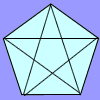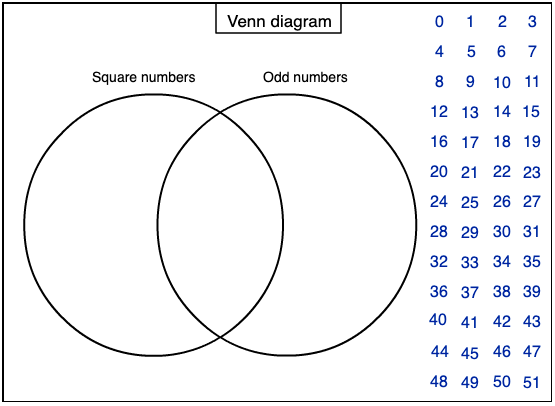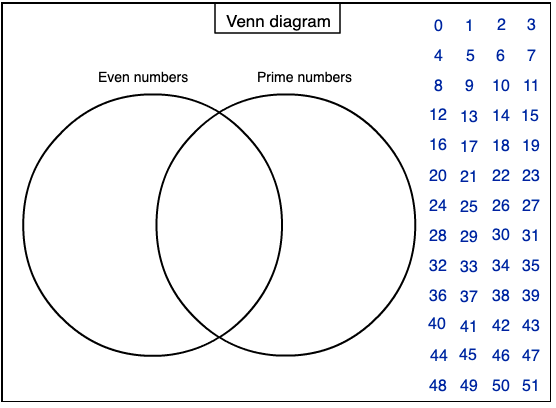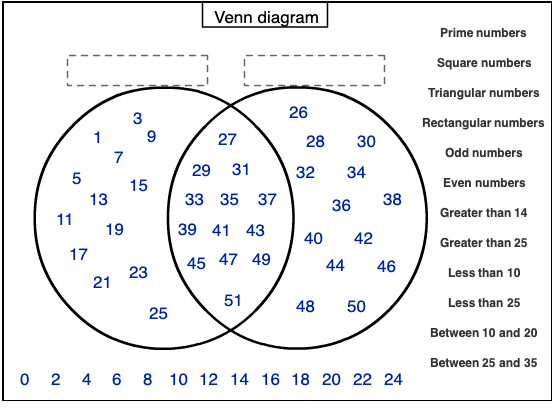#### You may also like### Number Detective

Follow the clues to find the mystery number.### Prime Magic

Place the numbers 1, 2, 3,..., 9 one on each square of a 3 by 3 grid so that all the rows and columns add up to a prime number. How many different solutions can you find?### Diagonal Trace

You can trace over all of the diagonals of a pentagon without lifting your pencil and without going over any more than once. Can the same thing be done with a hexagon or with a heptagon?

# Various Venns

## Various Venns

Can you place the numbers from 0 to 51 into this Venn diagram?How do you know where to put each number?
You might like to print off this sheet.

Here is another one for you to try.Again, you might like to print off this sheet.

The third Venn diagram below is a little different. This time you need to decide what sets the two groups represent. Choose the labels from the list on the right.Here is a sheet you can print.

Can you explain how you worked it out?

### Why do this problem?

The first two parts of this problem provide an opportunity for learners to become familiar with Venn diagrams, whilst reinforcing knowledge of number properties. The final part introduces slightly higher-level thinking as learners then work "backwards".

### Possible approach

If the group is not familiar with Venn diagrams, you could project the first image on an interactive whiteboard, and ask everyone to watch in silence as you place a few numbers in the correct regions. Invite the class to comment on what they notice and what they wonder, and use the ensuing discussion to clarify the representation.

If learners work on this task in pairs it will encourage them to construct mathematical arguments to convince each other where on the diagram each number belongs. Explaining out loud in this way often helps to clarify thinking and will give a purpose for accurate use of mathematical vocabulary.

As they work, listen out for any numbers or number properties that prove more tricky than others, and/or any pairs of learners that are reasoning clearly. You could draw attention to these in the final plenary.

### Key questions

Where would you put a number which is square but not odd?
Where would a square odd number go?
Where would a number which is odd but not square go?
Where would you put a number which is not square and not odd?

### Possible extension

Learners could try their hands at placing numbers in a Venn diagram with three circles. They could use three categories such as 'multiples of 7', 'square numbers' and 'odd numbers'. This sheet might be useful for this purpose.

### Possible support

You could try using this simpler version instead which uses number properties that children usually encounter before those contained in this problem.